Next: CAIN functions Up: Basic Grammer of the Previous: Commands

## Expressions

In the example in Sec.1.1, the right_hand_sides of some operands are written in the form of mathematical expressions. In general, `expression' is a mathematical expression which is almost identical to FORTRAN floating expression. It may contain
1. Literal numbers, such as 2, 2.0, -3E-5, etc.
To indicate the exponent, any of E,e,D,d,Q,q may be used. Note that there is no integer expression so that 2 is identical to 2.0.
2. Operators +,-,*,/,^. Note that power is indicated by ``^'' instead of ``**''.
3. Parenthesis: ( [ { ) ] } . Must match.
4. Pre-defined parameters : There are three types of predefined parameters. The first type is the universal constants that never change:
Pi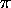E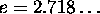Euler
Euler's constant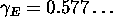Deg
0.0174=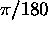. You can write, e.g., 10*Deg where the randian unit is required.
Cvel
velocity of light (m/sec).
Hbar
Planck's constant (Joulesec).
Hbarc
Planck's constant times the velocity of light (eVm).
Emass
Electron mass (eV/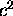).
Echarge
Elementary charge (Coulomb).
Reclass
LambdaC
Compton wavelength (m).
FinStrC
Fine structure constant.

The second type is the parameters whose values are determined by the program. Users cannot change their values but can refer to.
T,X,Y,S
Running variables  for particle coordinate (m).
En,Px,Py,Ps
Running variables for energy-momentum (eV, eV/c). The energy is En but not E.
Sx,Sy,Ss
Electron/positron spin. Helicity may be written approximately as Ss*Sgn(Ps).
Xi1,Xi2,Xi3
Photon Stokes parameters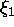,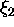,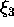.
Kind
Particle species. 1,2,3 for photon, electron, positron.
Gen
Particle generation.
Time
Running variables for global time coordinate (m).

The third type is those whose names are predefined with default values and which the user can change (by SET command) such as
MsgFile
File reference number for echo, error messages, and default destination of PRINT command. (default=6)Outfile
File reference number for voluminous outputs. The default destination of WRITE command. (default=12)
OutFile2
Other print output. Not used. (default=12)
TDFile
TopDrawer file number. (default=8)
MsgLevel
Message level. (default=0, i.e., error messages only)
Rand
Random number seed. (default=3)
Debug
Debug parameter for the programmer. If you set Debug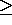2, call and return from major subroutines are announced. (default=0)
Smesh
Longitudinal mesh size  (m) for the calculation of beam-beam field, luminosity, etc. No default value.

5. User-defined parameters : Those defined by SET command. Upto 16 characters consisting of upper/lower case alphabets, numericals, and underscore `&#;'137'. The first character must not be a numerical.
6. Predefined functions  such as
I nt,Nint,Sgn,Step,Abs,Frac,Sqrt,Exp,Log,Log10,
C os,Sin,Tan, ArcSin,ArcCos,ArcTan,
C osh,Sinh,Tanh, ArcSinh,ArcCosh,ArcTanh, Gamma,
M od, Atan2, M in, Max
Defintions are the same as in standard FORTRAN except Sgn and Step:
to 10mmSgn(x)=1, 0, -1, for x>0, x=0, x<0.
to 10mmStep(x)=1, 0, for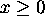, x<0.
Enclose the argument by ( ) or [ ] or { }. Separate arguments by ``,'' if there are more than one argument (Mod, Atan2, Min, Max). (Number of arguments for Min and Max is arbitrary.)
There are functions of other type, which are defined for CAIN. See the next subsection Sec.2.5.Next: CAIN functions Up: Basic Grammer of the Previous: Commands

Toshiaki Tauchi
Thu Dec 3 17:27:26 JST 1998